# create meme in R

I developed a tiny toy package, `meme`, which is now on CRAN. As it’s name indicated, it was designed to create memes, which are captioned photos that are intended to be funny, riduculous.

## `meme()`

The package is quite simple. You can use `meme()` function to add meme captions, and this is all the package supposed to do:

``````library(meme)
u <- "http://www.happyfamilyneeds.com/wp-content/uploads/2017/08/angry8.jpg"
meme(u, "code", "all the things!")
``````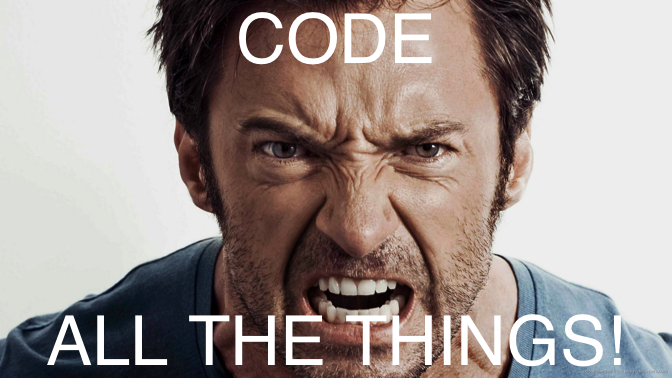## the grammar 🙈

The `meme` package was implemented using `grid` graphic system. Since `grid` is the most flexible graphic system in R, I try to mimic `ggplot2` (although very superficial) for practice.

User can use `mmplot()` to read and plot the input image and then using `+ mm_caption()` to add meme captions.

``````mmplot(u) + mm_caption("calm down", "and RTFM", color="purple")
``````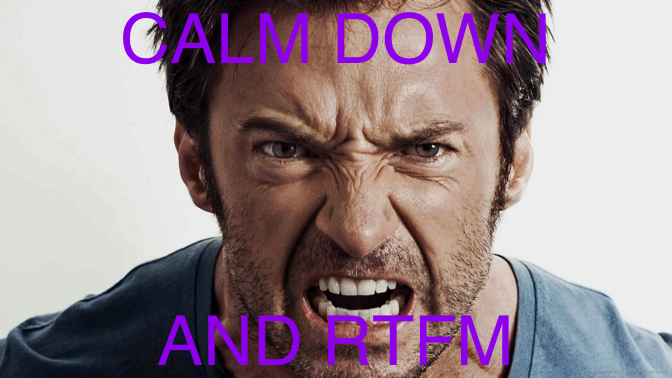## `meme_save()`

The `meme` output can be saved as an object, and can be exported to file using `ggsave()`. Since we would like to keep the original figure aspect ratio for output meme figure, I provide a helper function, `meme_save()`, which takes care of the figure aspect ratio and then called `ggsave()` to export the figure.

``````u2 <- "http://i0.kym-cdn.com/entries/icons/mobile/000/000/745/success.jpg"
x <- meme(u2, "please", "tell me more")
meme_save(x, file="docs/Figs/meme.png")
``````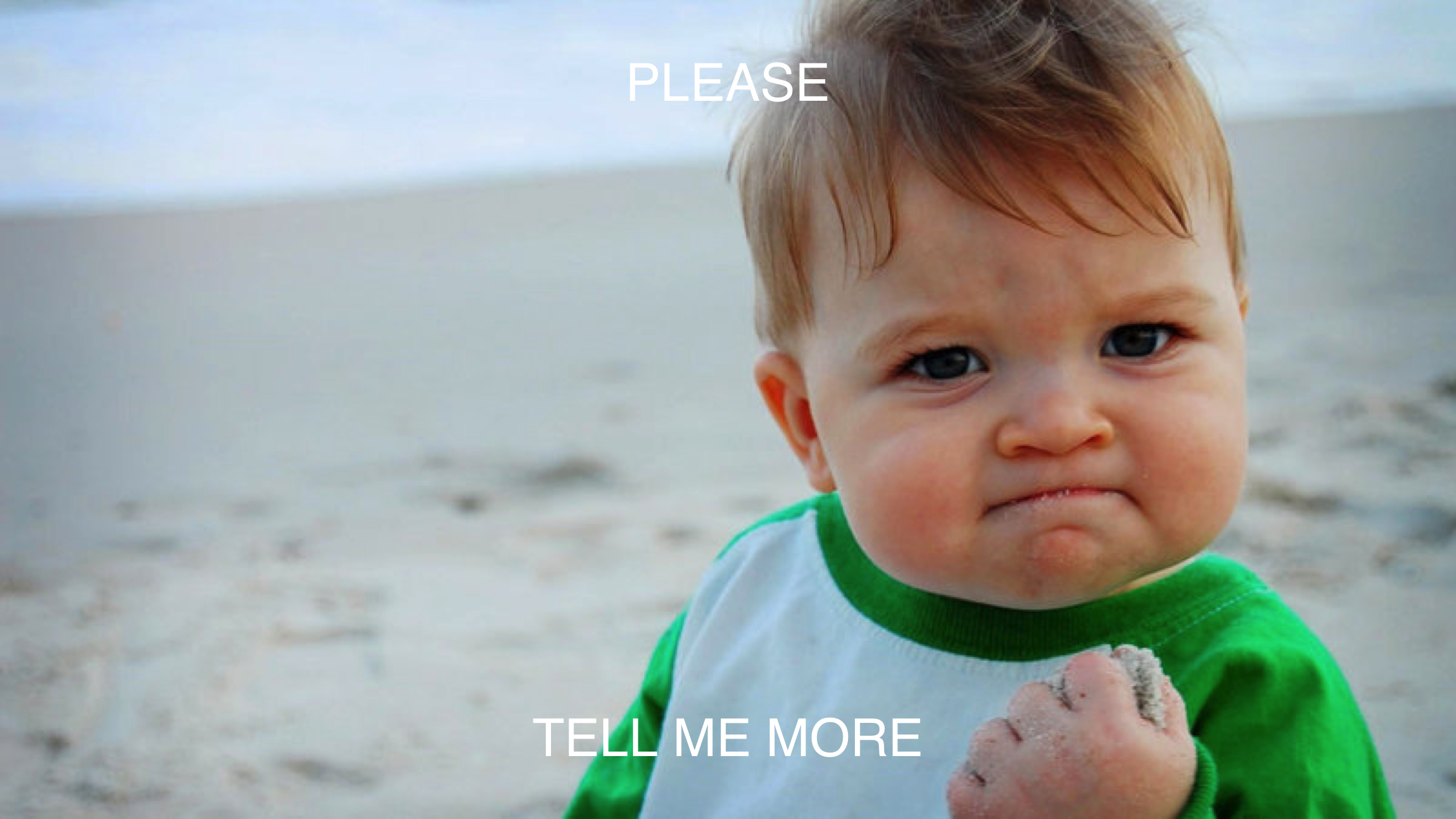## the `plot` method

Users can `plot` the `meme()` output and change the caption or other parameters in real time.

``````plot(x, size = 2, "happy friday!", "wait, sorry, it's monday", color = "firebrick", font = "Courier")
``````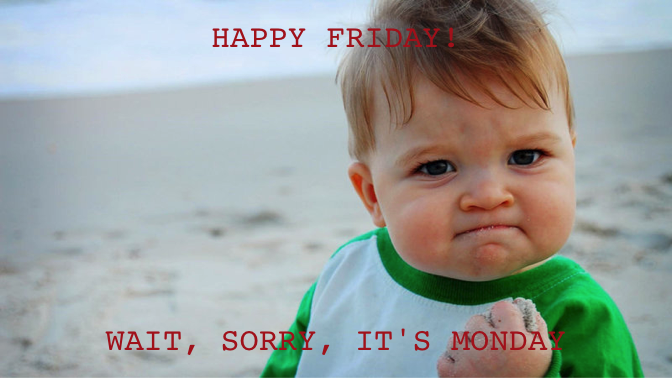## the `+` method

Instead of using parameters in `plot()` explictely, Users can use `+ aes()` to set the plot parameters:

``````x + aes(upper = "#barbarplots",
lower = "friends don't let friends make bar plots",
color = firebrick, font = Courier, size=1.5)
``````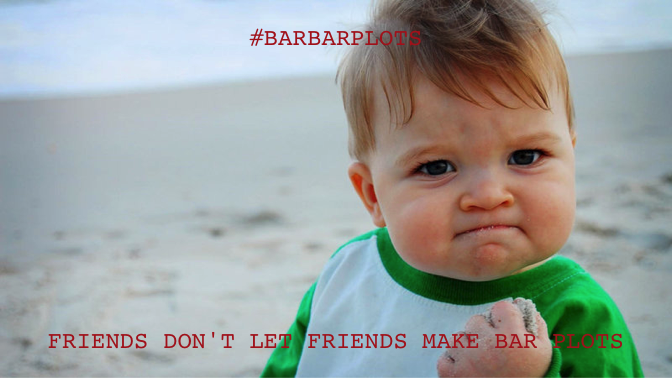or using `+ list()`. The following command will also generate the figure displayed above.

``````x + list(upper = "#barbarplots",
lower = "friends don't let friends make bar plots",
color = "firebrick", font = "Courier", size=1.5)
``````

## multi-language support

I didn’t do anything about it. Multi-language was supported internally. Just simply select a font for your language.

``````y <- meme(u, "卧槽", "听说你想用中文", font="STHeiti")
y
``````As the `meme` package was developed using `grid`, It would be better to provide function to convert the output object to `grob`. Similar to `ggplotGrob()` for `ggplot` object, I provide `memeGrob()` for the `meme` object and this making it possible to edit the details of the graph and compatible with the `grid` ecosystem.

Here are the examples of using `meme` in `grid`, `ggplot2` and `cowplot`.

## grid support

``````library(grid)
mm <- meme(u, "code", "all the things!", size=.3, color='firebrick')

grid.newpage()
pushViewport(viewport(width=.9, height=.9))
grid.rect(gp = gpar(lty="dashed"))

xx <- seq(0, 2*pi , length.out=10)
yy <- sin(xx)

for (i in seq_along(xx)) {
vp <- viewport(x = xx[i]/(2*pi), y = (yy[i]-min(yy))/2, width=.05, height=.05)
print(mm, vp = vp)
}
``````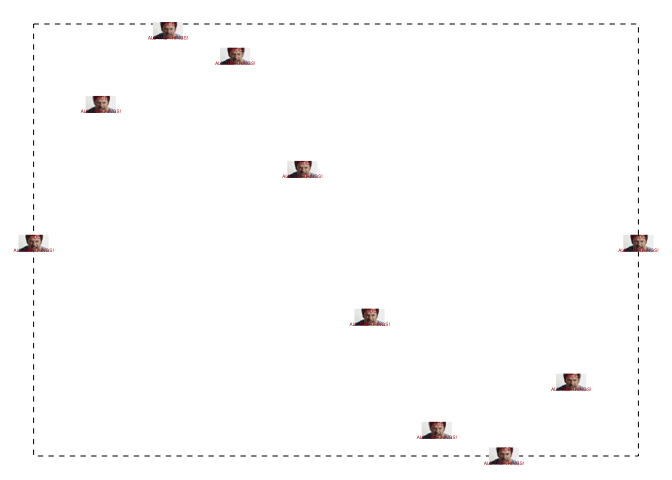## ggplot2 support

``````library(ggplot2)
library(ggimage)

d <- data.frame(x = xx, y = yy)
ggplot(d, aes(x, y)) + geom_line() +
geom_subview(mm, x = xx, y = yy, width=.3, height=.15)
``````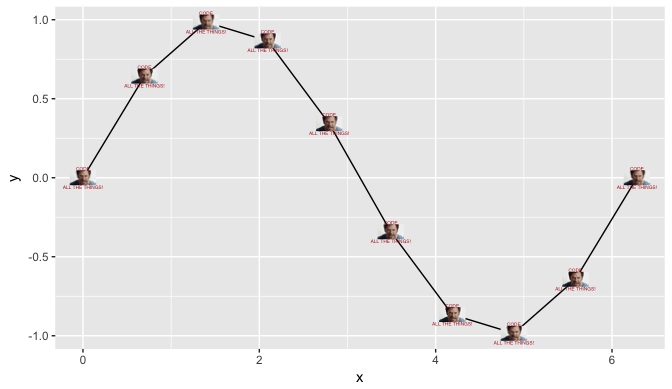``````ggplot(d, aes(x, y)) +
geom_subview(mm+aes(size=3), x=0, y=0, width=Inf, height=Inf) +
geom_point() + geom_line()
``````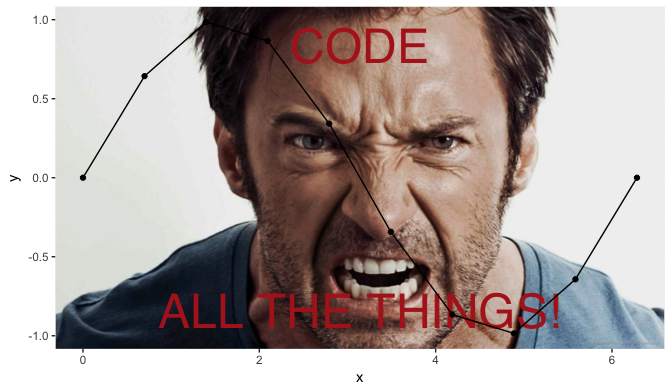## cowplot support

``````cowplot::plot_grid(x, y, ncol=1, labels = c("A", "B"))
``````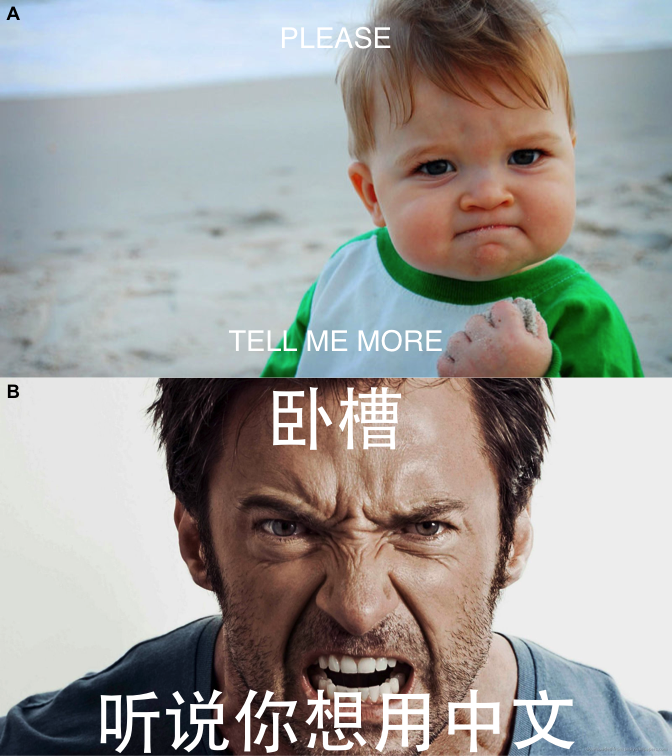Plea to against BMC
comments powered by Disqus